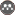Volume 15, Issue 56 (sumer 2011)                   JWSS 2011, 15(56): 181-191 | Back to browse issues page

BibTeX | RIS | EndNote | Medlars | ProCite | Reference Manager | RefWorks
Send citation to:V. R. Jalali, M. Homaee. Introducing a Nonparametric Model Using k-Nearest Neighbor Technique for Predicting Soil Bulk Density. JWSS. 2011; 15 (56) :181-191
URL: http://jstnar.iut.ac.ir/article-1-1717-en.html
, mhomaee@hotmail.com
Abstract:   (24870 Views)
Soil bulk density measurements are often required as an input parameter for models that predict soil processes. Nonparametric approaches are being used in various fields to estimate continuous variables. One type of the nonparametric lazy learning algorithms, a k-nearest neighbor (k-NN) algorithm was introduced and tested to estimate soil bulk density from other soil properties, including soil textural fractions, EC, pH, SP, OC and TNV. As many as eight nearest neighbors, based on cross validation technique were selected to perform bulk density prediction from the attributes of 136 soil samples. The nonparametric k-NN technique mostly performed equally well using Pearson correlation coefficient (r=0.86), root-mean-squared errors (RMSE=2.5) maximum error (ME=0.15), coefficient of determination (CD=1.3), modeling efficiency (EF=0.75) and coefficient of residual mass (CRM=0.001) statistics. It can be concluded that the k-NN technique is an alternative to other techniques such as pedotransfer functions (PTFs).